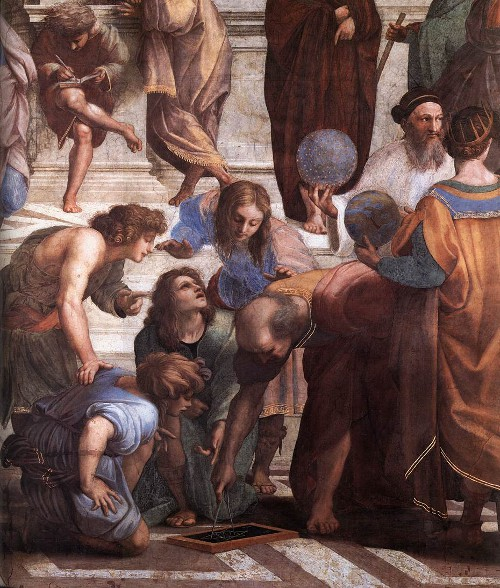# On Being Perfect (Numbers)

A number is called perfect if it is precisely equal to the sum of all the numbers that divide it exactly (excluding the number itself). In this sense, it's neither too large nor too small; it's just "perfect."
This post was published on the now-closed HuffPost Contributor platform. Contributors control their own work and posted freely to our site. If you need to flag this entry as abusive, send us an email.

A number is called perfect if it is precisely equal to the sum of all the numbers that divide it exactly (excluding the number itself). In this sense, it's neither too large nor too small; it's just "perfect." The first perfect number is 6, because 6 = 1 + 2 + 3, and the next one is 28 = 1 + 2 + 4 + 7 + 14.

While we don't know with certainty when perfect numbers were first defined, we do know that the Pythagoreans of the fifth century BCE were fascinated by them, because of their perceived "mystical" characteristics. Those sentiments propagated even into early Christianity. St. Augustine, for instance, wrote in the fifth century, "Six is a number perfect in itself, and not because God created all things in six days; rather, the converse is true. God created all things in six days because the number is perfect."

Similarly, the lunar cycle of 28 days was also taken as a heavenly phenomenon that conforms to a perfect number. The 28 Chinese "Mansions" (Ershiba Xiu) were the equivalent of the Western Zodiac constellations, dividing the Zodiacal band according to the lunar movement.

Remarkably, Euclid (Fig. 1) proved around 300 BCE that if, for some value of n, 2 − 1 is a prime number (divisible only by 1 and itself), then the number obtained from the product 2(2 − 1) is perfect. For instance, 2 − 1 = 8 − 1 = 7, which is a prime number. Therefore 2(2 − 1) = 4 × 7 = 28 is perfect. The great mathematician Leonhard Euler proved in the 18th century that all the even perfect numbers must be of the form suggested by Euclid.Figure 1. Euclid, as depicted in Raphael's painting "School of Athens."

The next two perfect numbers, 496 and 8,128, were also known in antiquity. The Greek mathematician Nicomachus of Gerasa noted the latter number around 100 CE. He was also the first person to write extensively about the perceived relationship between music and the workings of the cosmos -- the harmonic concept of the "music of the spheres."

A few facts about perfect numbers are absolutely astounding. First, even though the ancient Greeks already knew of the first four perfect numbers, the fifth -- 33,550,336 -- was first recorded thousands of years later, in a medieval manuscript. In fact, even today, we only know of 48 perfect numbers! The last of these, which has 34,850,340 digits, was discovered in February 2013 through the Great Internet Mersenne Prime Search (GIMPS) project, which has been running for 17 years. The second curiosity is that we still don't know whether odd perfect numbers exist (no one has ever found one). In fact, that is one of the unsolved problems in number theory. Nevertheless, even though mathematicians have not managed to discover any odd perfect numbers, they know of several stringent conditions that they must satisfy (if they exist). For instance, they must be greater than 10, and they must have at least 101 prime factors (and at least 12 distinct prime factors, if 3 is not one of the factors).

I guess that, as the name of these numbers implies, being perfect is hard, and it is rare. Large perfect numbers occur less and less frequently. Is there an infinite number of them? Nobody knows.

Close